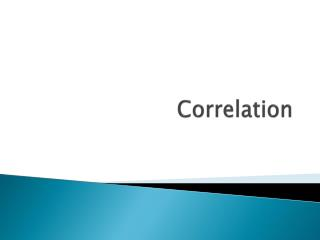# Correlation - PowerPoint PPT PresentationDownload PresentationCorrelation

CorrelationDownload Presentation## Correlation

- - - - - - - - - - - - - - - - - - - - - - - - - - - E N D - - - - - - - - - - - - - - - - - - - - - - - - - - -
##### Presentation Transcript

1. Correlation

2. Correlation Coefficient (r) Quantitative assessment of the strength of the relationship between x & y. It is the measure of the extent to which x & y are linearly related. *It is strong if the points lie close to a straight lint and is weak if they are widely scattered about a line.

3. Correlation (r) • It’s a number between -1 and 1. • The closer it is to the ends, the stronger the positive or negative relationship. • It gives the strength and direction. Strong Negative No Correlation Strong Positive -1 1 0

4. Let’s explore…. • www.whfreeman.com/tps4e

5. Pearson’s Correlation Coefficient Think about z-scores of the x & y

6. Pearson’s Correlation Coefficient Think about z-scores of the x & y

7. Pearson’s Correlation Coefficient Think about z-scores of the x & y

8. Find the correlation coefficient.

9. Find correlation coefficient.

10. AP Exam Tip If you are asked to interpret a correlation start by looking at a scatterplot of the data. Then be sure to address direction, form, strength, and outliers and put your answer in context.

11. Properties of “r” • It does not depend on the unit of measurement. • It doesn’t depend upon which of the 2 variables is “x” • It’s between -1 and 1 • It equals -1 or 1 if all points lie on a straight line • It is strongly affected by a few outliers

12. Scatterplots show relationships – not cause and effect.

13. Try One! • Create scatterplot • Find the correlation • Describe the association

14. Homework • Page 160 (14-18, 21, 26)